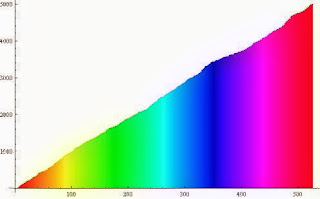## Friday, 29 November 2013

### A004782(n)=A014847(n)+1

﻿﻿﻿
﻿﻿A004782

The sequence of numbers where $latex \frac{2(2n-3)!}{n!(n-1)!}$ is an integer, is closely related to numbers n such that n-th Catalan number $latex \frac{C(2n,n)}{(n+1)}$ is divisible by n.
Theorem:
A004782(n)=A014847(n) + 1
Proof:

References:
- R. K. Guy, The On-Line Encyclopedia of Integer Sequences. A004782: 2(2n-3)!/n!(n-1)! is an integer.
- N. J. A. Sloane., The On-Line Encyclopedia of Integer Sequences. A014847: Numbers n such that n-th Catalan number C(2n,n)/(n+1) is divisible by n.
- N. J. A. Sloane., The On-Line Encyclopedia of Integer Sequences. A000984: Central binomial coefficients: C(2*n,n) = (2*n)!/(n!)^2.